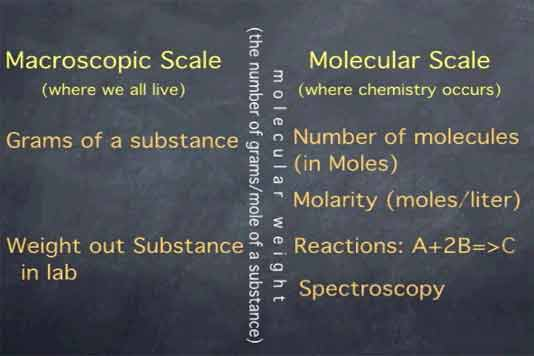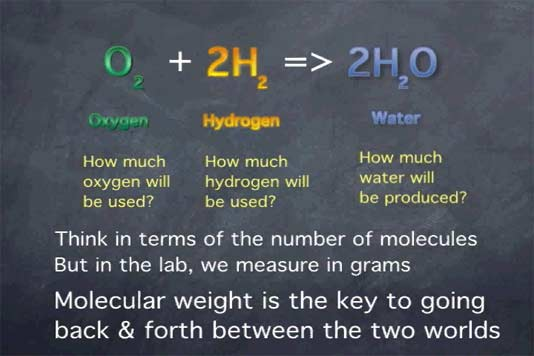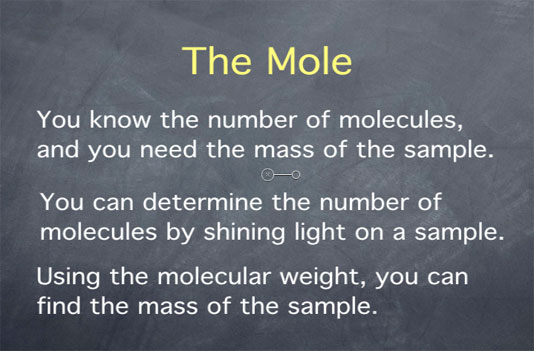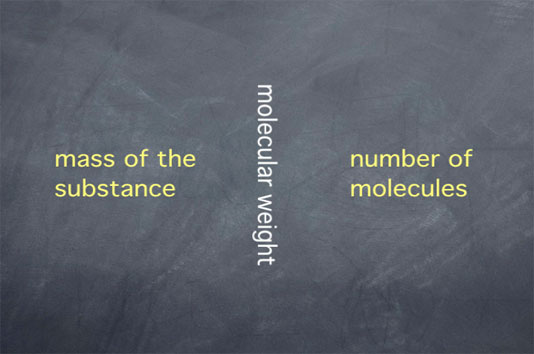# Stoichiometry Tutorials: The Mole

(from a complete OLI stoichiometry course)

You probably remember the mole from high school chemistry, but do you remember why it is useful to chemists? The goal of the following video is to give the "big picture" of the mole and its applications; information on how to use the mole in calculations can be found in another tutorial.

Throughout this course, we will use the term "molecular weight" to refer to the mass of a mole of a substance (for instance, the molecular weight of oxygen (O2) is 32 g/mol). Recent textbooks refer to this as "molar mass" to emphasize (i) that this term refers to the mass, not the weight, of substance, and (ii) that the quantity refers to a mole of a substance, not a single molecule. "Molecular weight" may be less precise, but it remains the term that most practicing chemists use in the laboratory. For this reason, we continue to use "molecular weight" in this course.

### The Mole Movie Text

The mole is one of the central concepts in stoichiometry. We will see it come up time and time again. The word "mole" refers to a specific number of molecules: 6.02 x 1023 molecules. This number is often refered to as "Avogadro's number." A mole is analogous to a dozen in that both refer to a specific number of items. A dozen eggs is 12 eggs, a dozen cars is 12 cars, and a dozen water molecules is 12 molecules.

A mole of eggs would be 6.02x1023 eggs, which is more eggs than you would ever see in everyday life. A mole of water molecules, however, the equivalent of 6.02x1023 water molecules, is about the amount in a small test tube of water. Just as the dozen is a useful quantity for counting eggs, the mole is a useful quantity for counting molecules.

The mole allows us to connect the macroscopic world, the surroundings we percieve, to the molecular scale, where chemistry occurs.On the macroscopic scale, we think in terms of grams of a substance. On the molecular scale, we think in terms of molecules, in moles. If a substance is in solution, we use molarity, which is moles per liter. The connection between these two realms is made with molecular weight.

The molecular weight is the number of grams per mole of a substance, or, less formally, how much a gram of the substance would weigh on the earth's surface. In calculations, this allows us to go back and forth between the amount of substance expressed in grams and the amount of substance expressed in number of molecules, as it is when you're dealing with moles.

So where is this useful?

When we weigh out a certain number of grams of a substance, we are working at the macroscopic scale. If we then perform a chemical reaction, that reaction occurs at the molecular scale. For instance, in the reaction: [see figure below]one molecule of oxygen reacts with two molecules of hydrogen to produce two molecules of water. If we want to understand how much oxygen or hydrogen will be consumed in a reaction, or how much water will be produced, we need to think in terms of the number of molecules of oxygen, hydrogen, and water. Conversely, in the laboratory, we must deal with grams of hydrogen, oxygen, and water. The molecular weight is the key to going back and forth between the macroscopic scale, where we weigh things, and the microscopic scale, where molecules react with one another.

Another place where the mole is useful is when you know the number of molecules and need to find the weight. For example, chemists will often shine light on a sample and see how much light it absorbs. From this, they can obtain the number of molecules.The mole has many other applications besides the ones we've touched on, which is why it is such an important concept to master. It appears, explicitly or implicitly, everywhere molecular weight must be related to the mass of a substance.Below you will find some practice questions to get you used to the concepts and calculations concerning the Mole.